Contents

foundations

# Contents

## Idea

Intuitionistic logic was introduced by Arend Heyting as a logic for Brouwer's intuitionistic mathematics. It applies more generally to constructive mathematics and so may also be called constructive logic.

Beware the terminological ambiguity: Some people insist that “intuitionistic logic” refers to Brouwerian intuitionism, which includes axioms that contradict classical logic; but other people use “intuitionistic” to mean the same as what in other contexts is called “constructive”, i.e. mathematics without the principle of excluded middle or the axiom of choice but nothing added that contradicts them. Some people (particularly material set theorists) use “constructive” to mean predicative constructive and “intuitionistic” to mean impredicative constructive.

## Idea

### General

Intuitionistic logic is most easily described as classical logic without the principle of excluded middle ($\vdash A \vee \neg{A}$) or the double-negation rule ($\neg\neg{A} \vdash A$). It may also be defined by starting with Gentzen's sequent calculus for classical logic (with $\neg$ but not $\bot$) and restricting to sequents $\Gamma \vdash \Delta$ where $\Delta$ may contain at most one formula, or by starting with sequent calculus with $\bot$ and restricting to such sequents where $\Delta$ must contain exactly one formula.

### Constructive interpretation of connectives

The intuitionistic interpretation of the logical connectives (known as the BHK interpretation, due to Kolmogorov (1932, p. 59), Heyting (1956, §7.1.1), Troelstra (1969, §2)) is such that the resulting proposition is regarded as true only if it is possible to construct a proof of its assertion.

For instance, to assert a logical conjunction (“and”) or a universal quantification (“for all”) is taken to mean to provide a proof of all the instances.

Dually but more notably, to assert a logical disjunction (“or”) or an existential quantification (“exists”) is taken to mean to prove one of the instances, so that there is no intuitionistic existence statement without construction of an example (the “disjunction property”, see below).

This constructive interpretation of logical truth is the crux of the rejection of the principle of excluded middle, for it implies that to prove $P \vee (\not P)$ (which may superficially/classically seem tautologous) one must prove $P$ or one must prove $\not P$ — but neither proof may be known (e.g. if $P$ = Riemann hypothesis).

(Here the classical mathematician is regarded as “idealistic” in their assumption that either case must hold, even if it is impossible to tell which one.)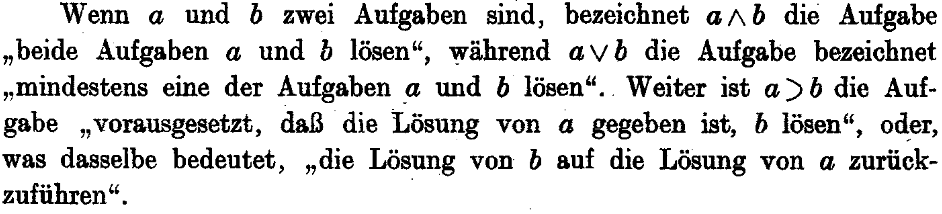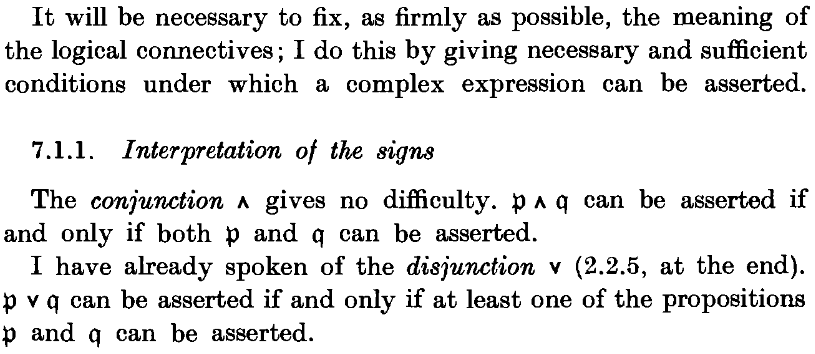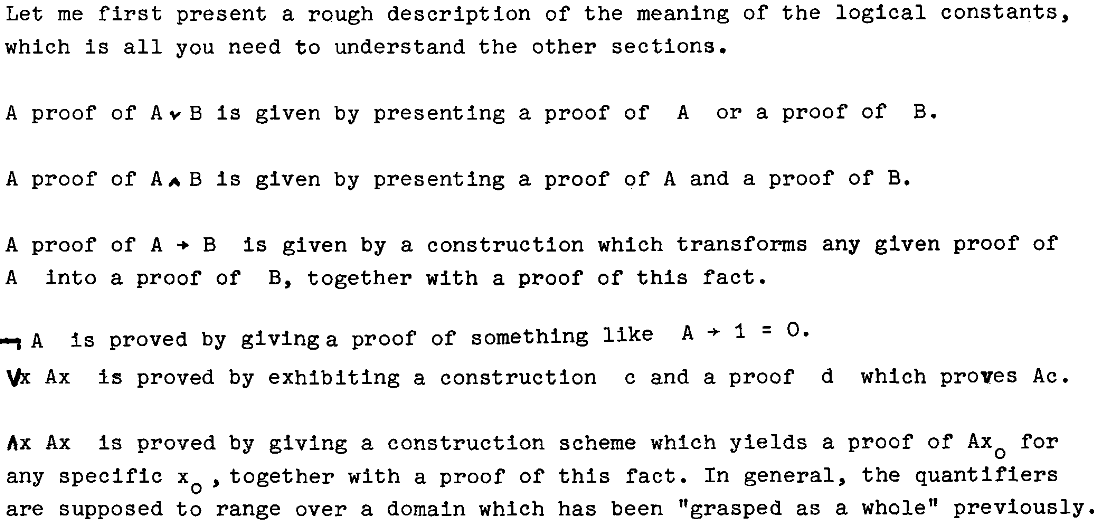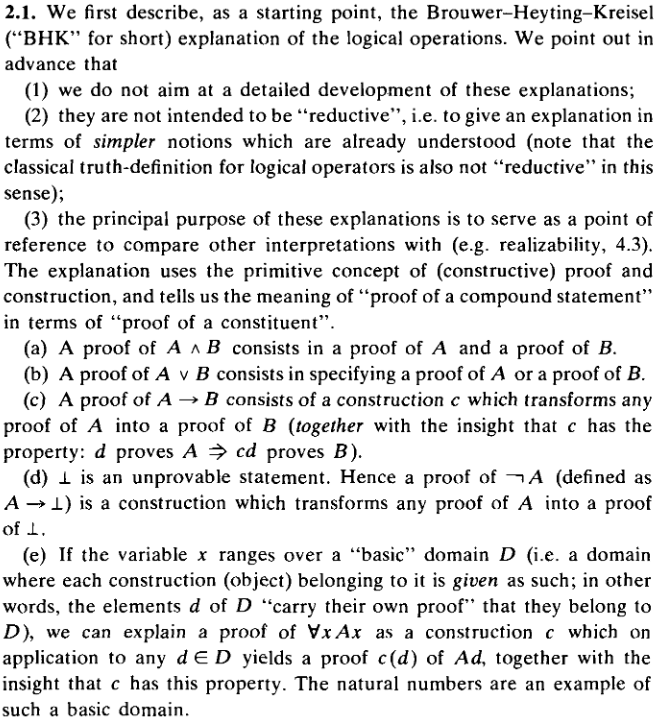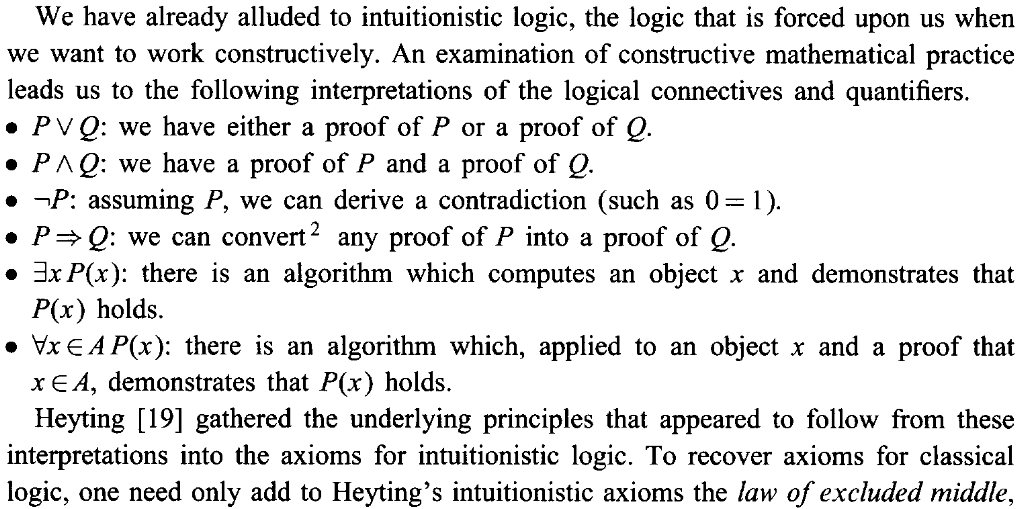The analogous discussion for inference rules in intuitionistic type theory is then given spring in Girard (1989, §2) and with more emphasis in Martin-Löf (1996, Lec 3).

## Properties

### Double negation

The double negation translation says that a proposition $P$ is provable in classical logic precisely if its double negation $\not \not P$ is provable in constructive logic.

### Disjunction property

Unlike classical logic, intuitionistic logic has the disjunction- and existence properties (cf. above): any proof of $\vdash A \vee B$ must contain a proof of either $\vdash A$ or $\vdash B$, and similarly any proof of $\vdash \exists x.\,F(x)$ must construct a term $t$ and a proof of $\vdash F(t)$. These properties are what justify our calling intuitionistic logic ‘constructive’.

On the other hand, (classical) Peano arithmetic is conservative over (intuitionistic) Heyting arithmetic when restricted to $\Pi^0_1$ formulas; that is, formulas of the form $\forall x\colon N.\, \exists y\colon N.\, F(x,y)$. Roughly speaking, classical logic can be just as ‘constructive’ as intuitionistic logic as far as proving the totality of functions $\mathbb{N} \to \mathbb{N}$ is concerned.

## Classicality principles

The principle of excluded middle is not provable in intuitionistic logic, and if we assume it then the logic becomes classical logic. But there are other principles that are provable classically but not intuitionistically, but which are weaker than full PEM, such as

Original articles on intuitionism:

Early monographs:

• Arend Heyting, Intuitionism: An introduction, Studies in Logic and the Foundations of Mathematics, North-Holland (1956, 1971) [ISBN:978-0720422399]

• Georg Kreisel, Section 2 of: Mathematical Logic, in T. Saaty et al. (ed.), Lectures on Modern Mathematics III, Wiley New York (1965) 95-195

Early historical account:

Recognizable statemens of the BHK interpretation of intuitionistic logic appear in

(who however speaks not of propositions but of Aufgaben, i.e. “tasks”, here in the sense of: “mathematical problems”)

(who is maybe the first to speak of the “meaning of logical connectives”)

and then in

(where it is presented as the author’s invention) and then in

(where the same is now called the “Brouwer-Heyting-Kreisel explanation”, not mentioning Kolmogorov)

and then in the context of intuitionistic type theory:

(where it is called Heyting semantics), further expanded on on:

• Per Martin-Löf, Lecture 3 of: On the Meanings of the Logical Constants and the Justifications of the Logical Laws, Nordic Journal of Philosophical Logic, 1 1 (1996) 11-60 [pdf, pdf]

and recalled in the context of constructive analysis: